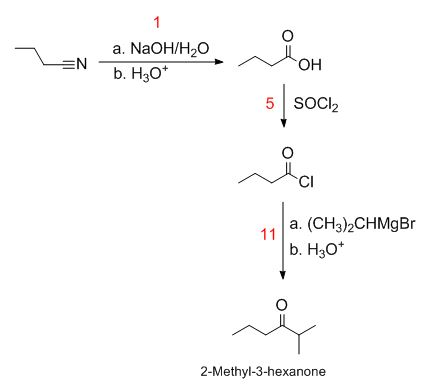# How would you synthesize the following compounds from butanenitrile using reagents from the...

## Question:

How would you synthesize the following compounds from butanenitrile using reagents from the table?

A. 2-Methyl-3-hexanone

B. Pentanoic acid

Reagents:

{eq}1.\\ a. NaOH/H_2O\\ b. H_3O^+\\ 2.\\ a. BH_3/THF\\ b. H_2O_2/NaOH\\ 3.PBr_3\\ 4. Dess-Martin \;periodinane \;in \; CHCl_2\\ 5. SOCl_2\\ 6. \\ a. LiAlH_4\\ b. H_3O^+\\ 7. NaCN\\ 8. CrO_3/H_3O^+\\ 9. \\ a. NaBH_4\\ b. H_3O^+\\ 10. \\ a. CH_3CH_2MgBr/dry\;ether\\ b.H_3O^+\\ 11.\\ a. (CH_3)2CHMgBr/dry\;ether\\ b.H_3O^+\\ 12. conc. \; HCl {/eq}

## Preparation of carboxylic acids:

One method of preparing carboxylic acids is through base catalyzed hydrolysis of nitriles followed by acidic workup. For example, butanoic acid can be prepared through reaction of butanenitrile with aqueous NaOH followed by acidic workup.

A. The synthesis of 2-methyl-3-hexanone from butanenitrile is shown below:Step 1: Hydrolysis of the nitrile using sodium hydroxide. The...

Become a Study.com member to unlock this answer! Create your account